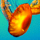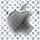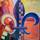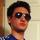## General Question# What does APR mean on credit card statements?

Asked by richill (2) August 15th, 2007

Interest rate numbers (i.e. 6%) seem to be different than the APR numbers.

Observing members: 0Composing members: 0APR is the cost of credit that consumers pay, expressed as a simple annual percentage.

xgunther (446)“Great Answer” (0) Flag as…annual percentage rate

gooch (5734)“Great Answer” (1) Flag as…xgunther and gooch are both right on your first question.

to address the second question, “interest rates” and “APRs” are usually different due to compounding (in simple english, compounding determines the way interest is charged on previous interest). with credit cards, “interest rate” is the rate that interest is charged on your unpaid balances—typically, this rate compounds monthly (though alternatives are possible, like daily). APR is the equivalent rate as if it compounded annually.

so for example, if you have a rate of 5.00% that compounds monthly, you actually have an APR of 5.12%. the extra .12% reflects how much interest you’ll actally pay in a year, because after you get charged interest at the end of the first month, in the second month you have to pay interest on the first month’s interest in addition to the regular interest you would have paid otherwise.

APR is supposed to give everyone a common base on which rates can be compared, because normal “interest rates” are meaningless unless you know how often they compound. and even if you know how often they compound, most people probably don’t know how to convert rates from one compounding to another.

FYI: if you want to do the calculation yourself, you can convert an interest rate (with monthly compounding) into an APR by using the following formula.

APR = ( (1 + ( interest rate / 12) ) ^ 12 ) – 1

SC (77)“Great Answer” (2) Flag as…or